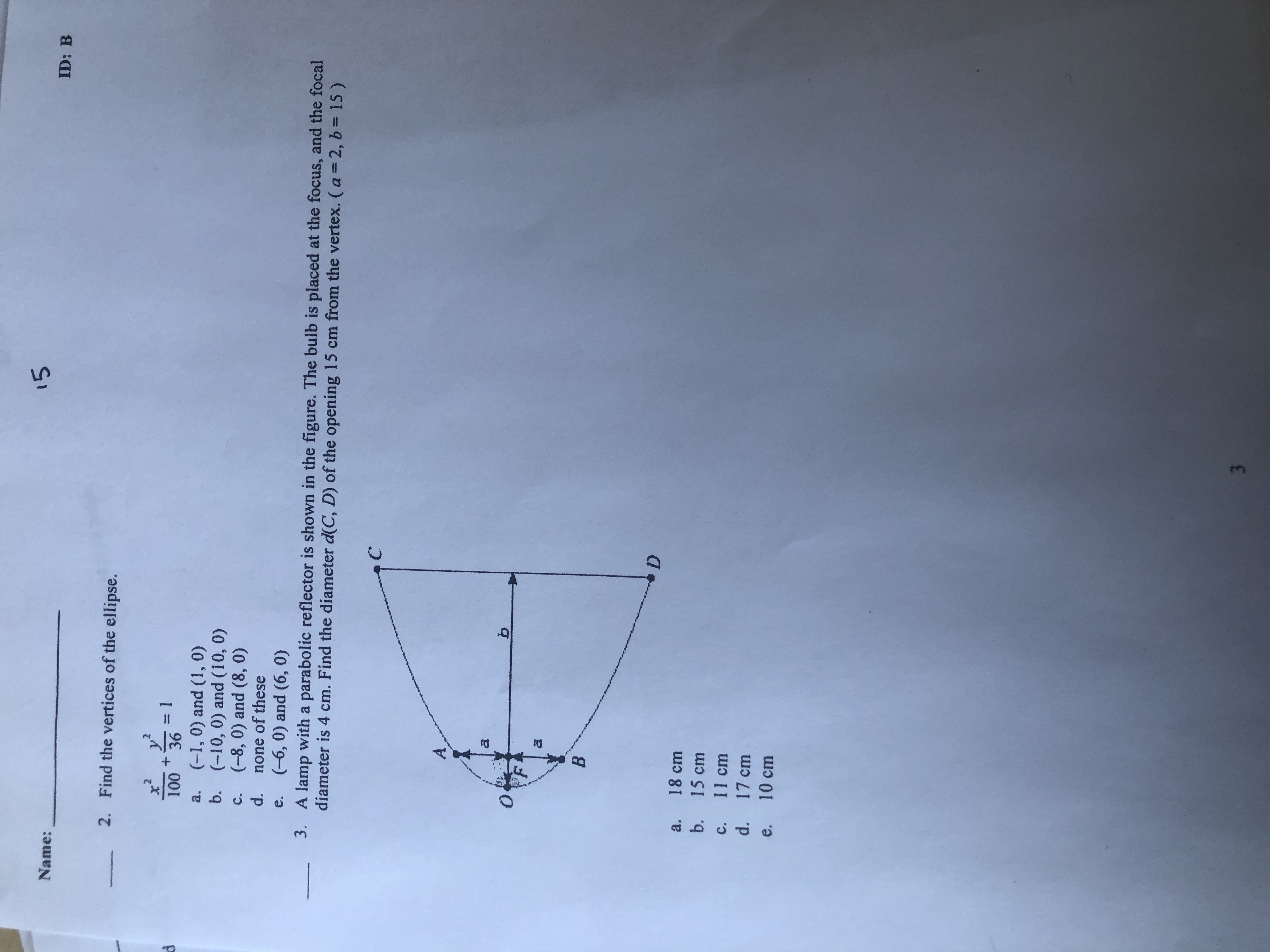# 15Name:ID: BFind the vertices of the ellipse.2.3D136100(-1, 0) and (1, 0)a.(-10, 0) and (10, 0)(-8, 0) and (8, 0)d.b.C.none of these(-6, 0) and (6, 0)e.3. A lamp with a parabolic reflector is shown in the figure. The bulb is placed at the focus, and the focaldiameter is 4 cm. Find the diameter d(C, D) of the opening 15 cm from the vertex. (a = 2, b = 15)18 cma.b.15 cm11 cmC.d. 17 cme. 10 cm3

Question
1 viewshelp_outlineImage Transcriptionclose15 Name: ID: B Find the vertices of the ellipse. 2. 3D1 36 100 (-1, 0) and (1, 0) a. (-10, 0) and (10, 0) (-8, 0) and (8, 0) d. b. C. none of these (-6, 0) and (6, 0) e. 3. A lamp with a parabolic reflector is shown in the figure. The bulb is placed at the focus, and the focal diameter is 4 cm. Find the diameter d(C, D) of the opening 15 cm from the vertex. (a = 2, b = 15) 18 cm a. b. 15 cm 11 cm C. d. 17 cm e. 10 cm 3 fullscreen
check_circle

Step 1

Given information

Focal diameter (4a) = 4 cm , distance of diameter d from vertex = 15 cm

Step 2

To determine

Diameter d of opening 15 cm from vertex

Step 3

Formula used

Standard equat...

### Want to see the full answer?

See Solution

#### Want to see this answer and more?

Solutions are written by subject experts who are available 24/7. Questions are typically answered within 1 hour.*

See Solution
*Response times may vary by subject and question.
Tagged in

### Calculus